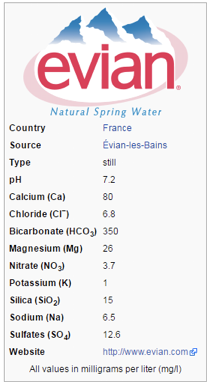# Analysis of the Evian water#

Written by Svetlana Kyas (ETH Zurich) on Mar 31th, 2022

This tutorial addresses the problem of checking the quality of Evian water using Reaktoro with a custom chemical composition.Evian water label, Source: wikipedia.org

We start by defining the chemical system with aqueous, gaseous and mineral phases, temperature, pressure and pH restrictions and their values:

from reaktoro import *
from math import *

db = PhreeqcDatabase("phreeqc.dat")

# Create an aqueous phase automatically selecting all species with provided elements
aqueousphase = AqueousPhase(speciate("C Ca Cl H K Mg N Na O S Si"))
aqueousphase.setActivityModel(chain(
ActivityModelHKF(),
ActivityModelDrummond("CO2"),
))

# Create a gaseous phase
gaseousphase = GaseousPhase("CO2(g)")
gaseousphase.setActivityModel(ActivityModelPengRobinson())

# Create mineral phases
minerals = MineralPhases()

# Create the chemical system
system = ChemicalSystem(db, aqueousphase, gaseousphase, minerals)

# Create constraints on temperature, pressure, and pH on the equilibrium chemical state
specs = EquilibriumSpecs(system)
specs.temperature()
specs.pressure()
specs.pH()

# Initialize the equilibrium solver
solver = EquilibriumSolver(specs)

# Define conditions to be satisfied at the chemical equilibrium state
conditions = EquilibriumConditions(specs)
conditions.temperature(25.0, "celsius")
conditions.pressure(1.0, "atm")
conditions.pH(7.2)


The composition of the initial chemical state is taken from the plot with Evian water label above. However, since the concentrations of the species in Evian water are defined as milligrams per liter (mg/L), we must convert these values to moles using the molar masses of the species. The latter can be determined with the function db.species("SpeicesName").molarMass(). To convert mg to kg, we multiply the values by 1e-6. We also equilibrate the chemical state with the given conditions.

# Initialize chemical state
state = ChemicalState(system)
state.set("H2O"    , 1.0, "kg")
state.set("Ca+2"   , 80 * 1e-6   / db.species("Ca+2").molarMass()   , "mol")  # 80 * 1e-6 kg / (MW(Ca++) kg / mol)
state.set("Cl-"    , 6.8 * 1e-6  / db.species("Cl-").molarMass()    , "mol")  # 6.8 * 1e-6 kg / (MW(Cl-) kg / mol)
state.set("HCO3-"  , 350 * 1e-6  / db.species("HCO3-").molarMass()  , "mol")  # 350 * 1e-6 kg / (MW(HCO3-) kg / mol)
state.set("Mg+2"   , 26 * 1e-6   / db.species("Mg+2").molarMass()   , "mol")  # 26 * 1e-6 kg / (MW(Mg++) kg / mol)
state.set("NO3-"   , 3.7 * 1e-6  / db.species("NO3-").molarMass()   , "mol")  # 3.7 * 1e-6 kg / (MW(NO3-) kg / mol)
state.set("K+"     , 1 * 1e-6    / db.species("K+").molarMass()     , "mol")  # 1 * 1e-6 kg / (MW(K+) kg / mol)
state.set("Na+"    , 6.5 * 1e-6  / db.species("Na+").molarMass()    , "mol")  # 6.5 * 1e-6 kg / (MW(Na+) kg / mol)
state.set("SO4-2"  , 12.6 * 1e-6 / db.species("SO4-2").molarMass()  , "mol")  # 12.6 * 1e-6 kg / (MW(SO4--) kg / mol)
state.set("SiO2(a)", 15 * 1e-6   / db.species("SiO2(a)").molarMass(), "mol")  # 15 * 1e-6 kg / (MW(SiO2) kg/ mol

# Equilibrate the solution with the given initial chemical state and desired conditions at equilibrium
res = solver.solve(state, conditions)


We then perform an analysis of the chemical equilibrium state of Evian water,i.e.,

• evaluation of the saturation indices of all the minerals contained in the database, which can be formed by chemical reactions and

• analysis of the composition of the solution.

To obtain saturation indices of minerals, we need to access the aqueous properties of the calculated chemical state. The saturation index is defined as log10 of the ratio of equilibrium constant and reaction quotient. It is 0 for minerals that are saturated or, in other words, precipitated and remain in equilibrium with the solution, SI > 0 for supersaturated minerals and SI < 0 for unsaturated minerals.

# Calculate saturation indices of selected minerals
props = AqueousProps(state)
print("SI (Calcite, CaCO3)       = ", props.saturationIndexLg('Calcite'))
print("SI (Aragonite, CaCO3)     = ", props.saturationIndexLg('Aragonite'))
print("SI (Dolomite, CaMg(CO3)2) = ", props.saturationIndexLg('Dolomite'))
print("SI (Quartz, SiO2)         = ", props.saturationIndexLg('Quartz'))
print("SI (Chalcedony, SiO2)     = ", props.saturationIndexLg('Chalcedony'))

SI (Calcite, CaCO3)       =  2.65391e-12
SI (Aragonite, CaCO3)     =  -0.14377
SI (Dolomite, CaMg(CO3)2) =  -0.0480374
SI (Quartz, SiO2)         =  -3.16124e-13
SI (Chalcedony, SiO2)     =  -0.429063


Based on the results above, the water is saturated with dolomite, whereas calcite and quartz are undersaturated.